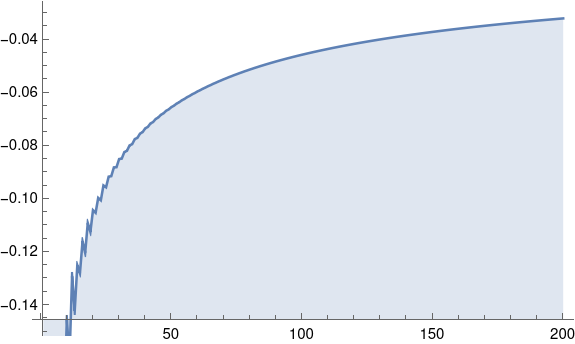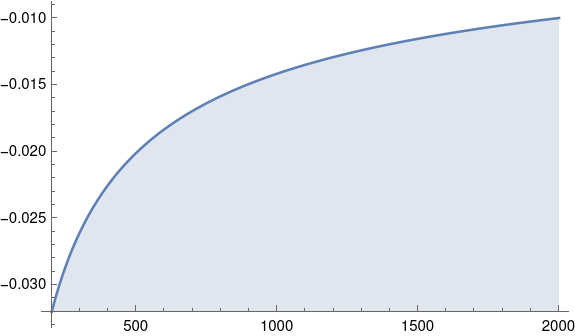# Estimating the number of integer partitions

Last week I wrote a few posts that included code that iterated over all partitions of a positive integer n. See here, here, and here. How long would it take these programs to run for large n?

For this post, I’ll focus just on how many partitions there are. It’s interesting to think about how you would generate the partitions, but that’s a topic for another time.

Ramanujan discovered an asymptotic formula for p(n), the number of partitions of n. As n increases,The ratio of the two sides of the equation goes to 1 as n → ∞, but how accurate is it for the sizes of n that you might want to iterate over in a program?

Before answering that, we need to decide what range of n we might use. Let’s be generous and say we might want to look at up to a trillion (1012) partitions. How big of a value of n does that correspond to? The estimate above shows p(200) is on the order of 4 × 1012, so we only need to be concerned with n ≤ 200. (See the next post for how to exactly solve for a n that gives a specified value.)

Here’s a plot to show the relative error in the approximation above.Here’s the Mathematica code that made the plot.

    approx[n_] := Exp[Pi Sqrt[2 n/3]]/(4 n Sqrt)
DiscretePlot[ 1 - approx[n]/PartitionsP[n], {n, 200}]


So the estimate above is always an under-estimate, at least about 4% low over the range we care about. It’s good enough for a quick calculation. It tells us, for example, to be very patient if we’re going to run any program that needs to iterate over all partitions of an integer even as small as 100.

The function PartitionsP above returns the number of partitions in its argument. It returned quickly, so it certainly did not generate all partitions and count them. Algorithms for computing p(n) might make an interesting blog post another time.

Even though it would be impractical to iterate over the partitions of larger values, we still might be curious what the plot above looks like for larger arguments. Let’s see what the plot would look like for n between 200 and 2000.The shape of the curve is practically the same.

The relative error dropped by about a factor or 3 when we increased n by a factor of 10. So we’d expect if we increase n by another factor of 10, the relative error would drop about another factor of 3, and indeed that’s what happens: when n = 20,000, the relative error is about -0.003.

## One thought on “Estimating the number of integer partitions”

1. Diethard Michaelis

The relative error cries for a log-scaled abscissa …